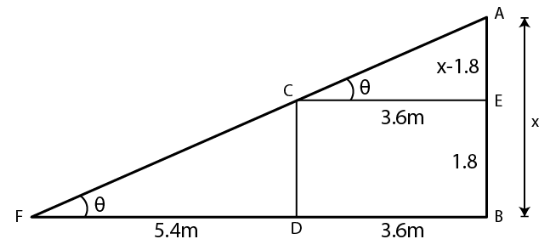Guru

# A man 1.8 m high stands at a distance of 3.6 m from a lamp post and casts a shadow of 5.4 m on the ground. Find the height of the lamp post.

• 2

sir this is the important  question from the book -ML aggarwal( avichal publication) class 10th , chapter20 , heights and distances
this is important question since is has been asked in previous year exam
A man 1.8 m high stands at a distance of 3.6 m from a lamp post
and casts a shadow of 5.4 m on the ground.
Find the height of the lamp post.

question no 31 , heights and distances , ICSE board

Share

1. Consider

AB as the lamp post

CD is the height of man

BD is the distance of man from the foot of the lamp

FD is the shadow of man

Construct CE parallel to DBTake AB = x and CD = 1.8 m

EB = CD = 1.8 m

AE = x – 1.8

In right triangle ACE

tan θ = AE/CE

Substituting the values

tan θ = (x – 1.8)/ 3.6 …… (1)

In right triangle CFD

tan θ = CD/FD

Substituting the values

tan θ = 1.8/5.4 = 1/3 ….. (2)

Using both the equations

(x – 1.8)/ 3.6 = 1/3

So we get

3x – 5.4 = 3.6

3x = 3.6 + 5.4 = 9.0

By division

x = 9/3 = 3.0

Hence, the height of lamp post is 3 m.

• 3